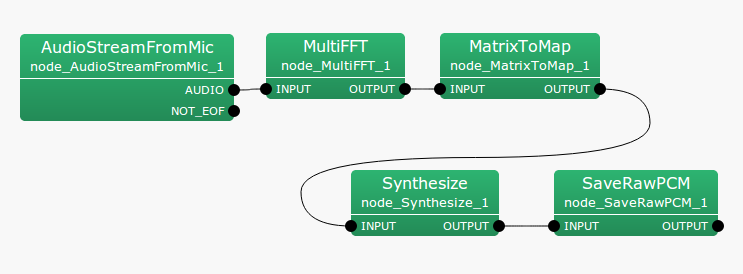## 6.7.14 Synthesize

### 6.7.14.1 Outline of the node

This node converts signals of frequency domain into waveforms of time domain.

### 6.7.14.2 Necessary files

No files are required.

### 6.7.14.3 Usage

This node is used to convert signals of frequency domain into waveforms of time domain.

When to useFigure 6.112: Example of a connection of Synthesize

### 6.7.14.4 Input-output and properties of the node

Table 6.83: Parameters of Synthesize
 Parameter name Type Default value Unit Description LENGTH 512 [pt] FFT length ADVANCE 160 [pt] Shift length SAMPLING_RATE 16000 [Hz] Sampling rate MIN_FREQUENCY 125 [Hz] Minimum frequency MAX_FREQUENCY 7900 [Hz] Maximum frequency WINDOW HAMMING Window function OUTPUT_GAIN 1.0 Output gain

Input

INPUT

Output

OUTPUT

Parameter

LENGTH

FFT length; must be equal to the other node (MultiFFT ).

Shift length; must be equal to the other node (MultiFFT ).

SAMPLING_RATE

Sampling rate; must be equal to the other nodes.

MIN_FREQUENCY

The minimum frequency used for waveform generation

MAX_FREQUENCY

The maximum frequency used for waveform generation

WINDOW

Window function. Select HAMMING, RECTANGLE or CONJ

OUTPUT_GAIN

Output gain

### 6.7.14.5 Details of the node

Inverse FFT is performed on four low bands of the input signals of the frequency domain by substituting 0 for frequency bins over $\omega _ s/2-100$ [Hz]. A designated window is adopted for overlap-add processing, a method of reducing the influence of a window by performing inverse transformation for every frame, adding the signals for which the time domains are shifted back while shifting them. For details, see the web pages in References. Finally, the temporal waveforms are multiplied by the output gain and output. Further, subsequent frames must be read for overlap-add processing; therefore, this node delays the entire processor. The length of the delay can be calculated as:

 \begin{equation} delay = \left\{ \begin{array}{@{\, }ll} \left| \mathrm{LENGTH}/\mathrm{ADVANCE} \right|- 1,& {if~ ~ } \mathrm{LENGTH} \, mod\, \mathrm{ADVANCE} \ne 0,\\ \mathrm{LENGTH}/\mathrm{ADVANCE}, & {otherwise}. \end{array} \right. \end{equation} (148)

Since the default values for HARK are LENGTH = 512 and ADVANCE = 160, the delay is three frames, which resulting in a 30 [ms] delay for the entire system.